• 在实际问题中，要研究两组多重相关变量间的相互依赖关系时，可使用的方法有：经典多元线性回归分析（MLR）、主成分回归分析（PCR）、偏最小二乘回归分析（PLS）。 当两组变量的个数很多，且都存在多重相关性，而观测...
在实际问题中，要研究两组多重相关变量间的相互依赖关系时，可使用的方法有：经典多元线性回归分析（MLR）、主成分回归分析（PCR）、偏最小二乘回归分析（PLS）。
当两组变量的个数很多，且都存在多重相关性，而观测的样本数又较少时，用PLS建立模型具有MLR等方法所没有的优点。
PLS在建模的过程中集中了主成分分析、典型相关分析、线性回归分析方法的特点，除了能提供一个合理的回归模型外，还可以提供一些更深入的信息。
1. 偏最小二乘回归分析概述
假定p个因变量$y_1,\cdots,y_p$与m个自变量$x_1,\cdots,x_m$均为标准化变量。自变量组合因变量组的标准化观测数据矩阵分别为$\bm{A}^{n\times m},\bm{B}^{n\times p}$，偏最小二乘回归分析建模的具体步骤如下：

分别提取两变量组的第一对成分$u_1,v_1$，代表自变量和因变量的线性组合$u_1=\bm{\rho^{(1)T}X}\ ,v_1=\bm{\gamma^{(1)T}Y}$为了回归分析的需要，要求：① $u_1$和$v_1$各自尽可能多地提取所在变量组的变异信息；② $u_1$和$v_1$的相关程度达到最大。
由两组变量集的标准化观测数据矩阵$\bm{A}$和$\bm{B}$，可以计算第一对成分的得分向量，记为$\bm{\hat{u}_1}$和$\bm{\hat{v}_1}$$\bm{\hat{u}_1=A\rho^{(1)}}\ ,\bm{\hat{v}_1=B\gamma^{(1)}}$此时可将以上两个要求化为数学上的条件极值问题$\max(\bm{\hat{u}_1\cdot\hat{v}_1})=\bm{\rho^{(1)T}A^TB\gamma^{(1)}}\\ \text{s.t.}\begin{cases}\bm{\rho^{(1)T}\rho^{(1)}}=||\bm{\rho^{(1)}}||^2=1\\\bm{\gamma^{(1)T}\gamma^{(1)}}=||\bm{\gamma^{(1)}}||^2=1\end{cases}$利用拉格朗日乘数法，将问题转为计算矩阵$\bm{M=A^TBB^TA}$的特征值和特征向量，其中最大特征值对应的单位特征向量就是$\bm{\rho^{(1)}}$，且有$\bm{\gamma^{(1)}}=\cfrac{1}{\lambda_1}\bm{B^TA\rho^{(1)}}$。
建立$y_1,\cdots,y_p$对$u_1$的回归，及$x_1,\cdots,x_m$对$u_1$的回归。假定回归模型为$\begin{cases}\bm{A=\hat{u}_1\sigma^{(1)T}+A_1}\\\bm{B=\hat{u}_1\tau^{(1)T}+B_1}\end{cases}$式中$\bm{\sigma^{(1)}}=[\sigma_{11},\cdots,\sigma_{1m}]^T\ ,\bm{\tau^{(1)}}=[\tau_{11},\cdots,\tau_{1p}]^T$分别为多对一的回归模型中的参数向量；$\bm{A_1,B_1}$是残差阵。则回归系数向量$\bm{\sigma^{(1)}},\bm{\tau^{(1)}}$的最小二乘估计为$\begin{cases}\bm{\sigma^{(1)}=A^T\hat{u}_1/||\hat{u}_1||^2}\\\bm{\tau^{(1)}=B^T\hat{u}_1/||\hat{u}_1||^2}\end{cases}$称$\bm{\sigma^{(1)},\tau^{(1)}}$为模型效应负荷量。
若残差阵$\bm{B_1}$中元素的绝对值近似为0，则认为用第一个成分建立的回归式精度已经满足需要了，可以停止抽取成分。否则用残差阵$\bm{A_1,B_1}$代替$\bm{A,B}$，重复以上步骤。得$\begin{cases}\bm{A=\hat{u}_1\sigma^{(1)T}+\hat{u}_2\sigma^{(2)T}+A_2}\\\bm{B=\hat{u}_1\tau^{(1)T}+\hat{u}_2\tau^{(2)T}+B_2}\end{cases}$
设$n\times m$数据阵$\bm{A}$的秩为$r\leqslant\min(n-1,m)$，则存在r个成分$u_1,\cdots,u_r$，使得$\begin{cases}\bm{A=\hat{u}_1\sigma^{(1)T}+\cdots+\hat{u}_r\sigma^{(r)T}+A_r}\\\bm{B=\hat{u}_1\tau^{(1)T}+\cdots+\hat{u}_r\tau^{(r)T}+B_r}\end{cases}$将$u\sim x$带入$y\sim u$，即得$y\sim x$的偏最小二乘回归方程。
交叉有效性检验：一般情况下，偏最小二乘法并不需要选用存在的r个成分$u_1,\cdots,u_r$来建立回归式，只选用前$l$个成分即可得到预测能力较好地回归模型。对于建模所需提取的成分个数$l$，可通过交叉有效性检验来确定。
每次舍去第$i(i=1,2,\cdots,n)$个观测数据，对余下n-1个观测数据用偏最小二乘回归方法建模，并考虑抽取$h(h\leqslant r)$个成分后拟合的回归式，然后把舍去的自变量组第i个观测数据代入所拟合的回归方程式，得到$y_j(j=1,2,\cdots,p)$在第i个观测点上的预测值$\hat{b}_{(i)j}(h)$。
对$i=1,2,\cdots,n$重复以上的验证，抽取h个成分时第j个因变量$y_j$的预测误差平方和为$\operatorname{PRESS}_j(h)=\sum_{i=1}^n[b_{ij}-\hat{b}_{(i)j}(h)]^2$$\bm{Y}=[y_1,\cdots,y_p]^T$的预测误差平方和为$\operatorname{PRESS}(h)=\sum_{j=1}^p\operatorname{PRESS}_j(h)$另外，再采用所有的样本点，拟合含h个成分的回归方程。此时，记第i个样本点的预测值为$\hat{b}_{ij}(h)$，则可以定义$y_j$的误差平方和为$\operatorname{SS}_j(h)=\sum_{i=1}^n[b_{ij}-\hat{b}_{ij}(h)]^2$定义$\bm{Y}$的误差平方和为$\operatorname{SS}(h)=\sum_{j=1}^p\operatorname{SS}_j(h)$当$\operatorname{PRESS}(h)$达到最小值时，对应的h即为所求得成分个数l。定义交叉有效性为$Q^2_h=1-\cfrac{\operatorname{PRESS}(h)}{\operatorname{SS}(h-1)}$在建模的每一步计算结束前，均进行交叉有效性检验，如果将限制值设为0.05，在第h步有$Q_h^2<1-0.95^2=0.0975$，则模型达到精度要求，可停止提取成分；否则表示第h步提取的$u_h$成分边际贡献显著，应继续第h+1步计算。

2. Matlab偏最小二乘回归命令plsregress
[XL,YL,XS,YS,BETA,PCTVAR,MSE,stats] = plsregress(X,Y,ncomp)


X为[n,m]的自变量数据阵；Y为[n,p]的因变量数据阵；ncomp为成分个数，默认为min(n-1,m)
XL为[m,ncomp]的负荷量矩阵$\bm{\sigma}$；YL为[p,ncomp]的负荷量矩阵$\bm{\tau}$；XS为$\bm{\hat{u}}$的得分矩阵；YS为$\bm{\hat{v}}$的得分矩阵
BETA的每一列对应$y\sim x$的回归表达式；PCTVAR是一个两行的矩阵，两行分别代表每个元素对应自变量和因变量提出成分的贡献率；MSE是一个两行的矩阵，两行分别代表自变量和因变量对应提出成分之间回归方程的剩余标准差
stats返回4个值，其中stats.W的每一列对应特征向量$\bm{\rho}$，Matlab算得的特征向量不是单位向量


展开全文数学建模
• 飞机起飞性能偏最小二乘回归分析，乔爽，肖攸安，为了提高飞机起飞性能实验的精确度，减少试验次数，降低试验成本，提出了在均匀设计后应用偏最小二乘法回归进行数据处理的方案。
• 介绍数学建模中偏最小二乘的应用，介绍偏最小二乘原理及项目案例代码。
• 偏最小二乘回归分析实例plsregress命令
偏最小二乘回归分析实例

原文：http://blog.csdn.net/qq_34861102/article/details/77102683

plsregress命令

步骤 数据标准化
求相关系数矩阵
分别提出自变量组合因变量组的成分
求两个成分对标准化指标变量和成分变量之间的回归方程
求因变量组与自变量组之间的回归方程
模型的解释与检验
实例代码

ab0 = load('data.txt');
%均值和方差
mu = mean(ab0);
sig = std(ab0);
%标准化以后的自变量和因变量数据
a = ab(:,[1:end-1]);
b = ab(:,end);
[XL YL XS YS BETA PCTVAR MSE status] = plsregress(a,b);
%求自变量的主成分系数
xw = a\XS;
%求因变量的主成分系数
yw = b\YS;
ncomp = input('请根据PCTVAR的值确定提出成分对的个数');

[XL2 YL2 XS2 YS2 BETA2 PCTVAR2 MSE2 status2] = plsregress(a,b,ncomp);
%自变量的个数
n = size(a,2);
%原始数据回归方程的常数项
beta3(1) = mu(end) - mu(1:n)./sig(1:n)*BETA2([2:end]).*sig(end);
beta3([2:n+1]) = (1./sig(1:n))'*sig(n+1:end).*BETA2([2:end]);
%直方图
bar(BETA2','k');
%y的预测值
yhat = repmat(beta3(1),[size(a,1),1]) + ab0(:,[1:n])*beta3([2:end])';
%预测值的最大值
ymax = max([yhat;ab0(:,end)]);

展开全文实例
• 偏最小二乘回归分析在建模过程中集中了主成分分析，典型相关分析和线性回归分 析方法的特点，因此在分析结果中，除了可以提供一个更为合理的回归模型外，还可以 同时完成一些类似于主成分分析和典型相关分析的研究...
• 讲得比较清晰的一个偏最小二乘，附带了MATLAB偏最小二乘函数的使用
• 为了科学预测试验装备修理成本,提高维修经费决策质量,引入偏最小二乘回归分析(Partial Least Squares Regression,PLSR)对试验装备修理成本进行预测。针对试验装备修理成本小样本、贫数据、特征量相关性强的不利条件,...
• 偏最小二乘回归(PLS-Partial Least Squares)是一种新型的多元统计数据分析方法，是一种多因变量对多自变量的回归建模方法，是对最小二乘方法的推广。 优点： 1）提供了一种多因变量对多自变量的回归建模方法； 2）...
EverydayOneCat
💯💯！知识点
1.偏最小二乘回归介绍
偏最小二乘回归(PLS-Partial Least Squares)是一种新型的多元统计数据分析方法，是一种多因变量对多自变量的回归建模方法，是对最小二乘方法的推广。
优点：
1）提供了一种多因变量对多自变量的回归建模方法；
2）有效地解决变量之间的多重共线性问题；
3）适合当样本点数量少于自变量个数时进行回归建模；
4）最终模型中含原有所有自变量，回归系数容易解释。
5）计算简单、预测精度高，易于定性解释。
偏最小二乘回归≈主成分分析+典型相关分析+多元回归
2.偏最小二乘回归法的建模
（1）建立回归方程进行共线性检验
（2）若存在共线性，进行偏最小二乘回归
（3）确定成分个数
（4）建立偏最小二乘回归模型，进行解释
例：某康复俱乐部对20名中年人测量了
三个生理指标：体重x1, 腰围x2, 脉搏x3
三个训练指标: 单杠y1, 弯曲y2, 跳高y3
试用偏最小二乘回归建立由三个生理指标分别预测三个训练指标的回归模型。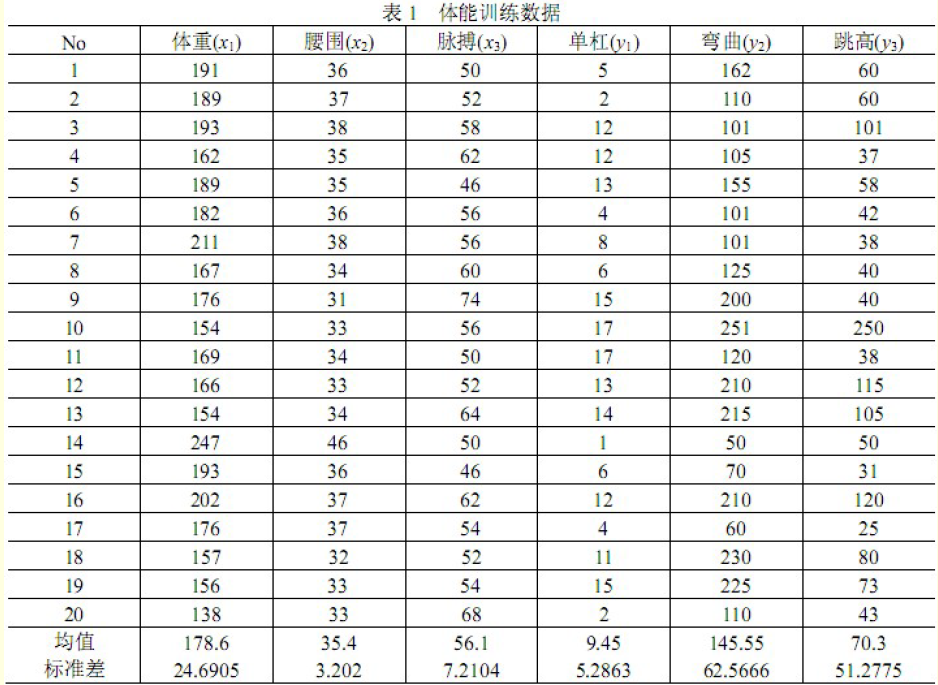SAS代码：
data example;
input x1-x3 y1-y3 @@;
cards; @@：/**/指针控制符，读取下面数据时自动换行
输入数据
；
proc corr data=example;  /*proc:过程步的开始，表示调用*/
var x1-x3 y1-y3;  /*corr:相关系数矩阵*/
run;
proc pls data=example cv=one details;/*pls: 偏最小二乘回归;cv=one:舍一交叉有效性检验*/
model y1-y3=x1-x3/solution; /*solution:标准化和原本的都有*/
run;

相关系数矩阵：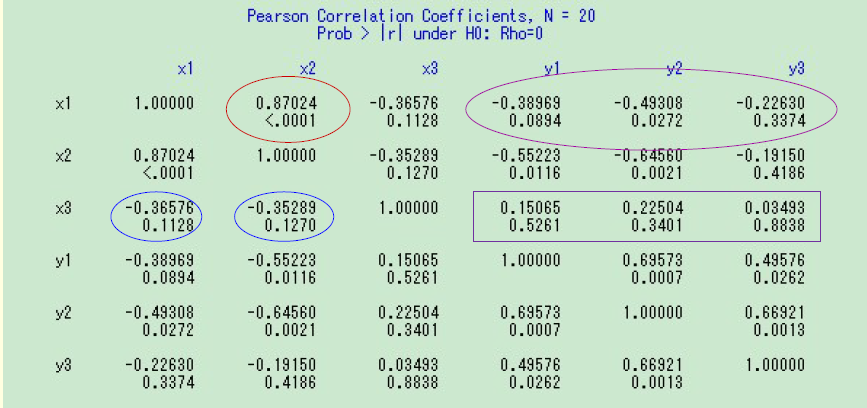1.体重和腰围正相关； 2.体重、腰围与脉搏负相关；
3.单杠、跳高、弯曲的训练成绩与体重、腰围负相关，与脉搏正相关
用交叉验证法确定提取成分个数：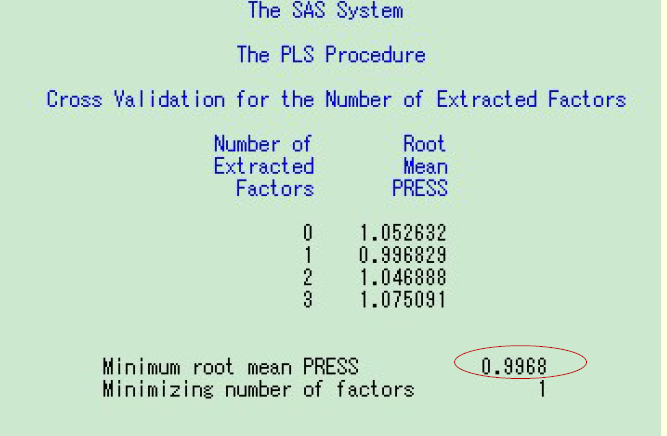由结果可知，采用舍一交叉验证法提取1个成分,可使得PRESS最小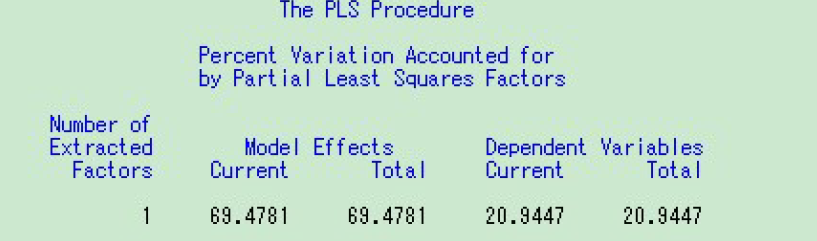提取的1个成分解释自变量的比率为69.4781%，解释因变量的比率为20.9447%。
标准化模型参数估计：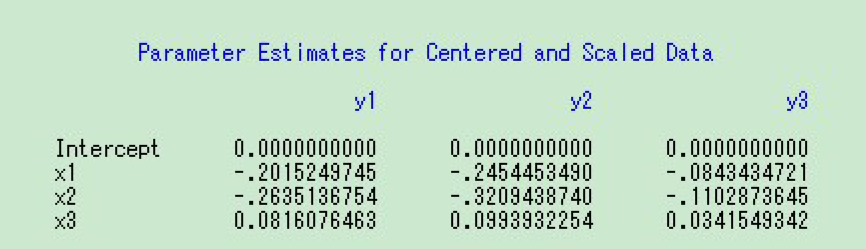由表可写出标准化变量的回归方程，结果如下：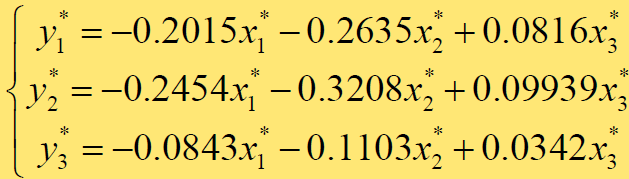原始模型的参数估计：还原成原始变量的回归方程，结果如下：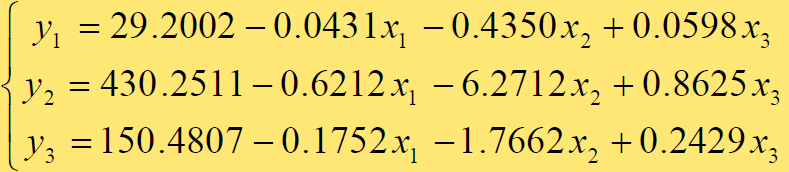作业
下列数据是2个因变量和6个自变量。请用偏最小二乘回归建立方程组，并预测最后四个数的因变量值。

Y1
Y2
X1
X2
X3
X4
X5
X6

1394.89
2505
519.01
8144
373.9
117.3
112.6
843.43

920.11
2720
345.46
6501
342.8
115.2
110.6
582.51

2849.52
1258
704.87
4839
2233.3
115.2
115.8
1234.85

1092.48
1250
290.90
4721
717.3
116.9
115.6
697.25

832.88
1387
250.23
4134
781.7
117.5
116.8
419.39

2793.37
2397
387.99
4911
1371.1
116.1
114.0
1840.55

1129.20
1872
320.45
4430
497.4
115.2
114.2
762.47

2014.53
2334
435.73
4145
824.8
116.1
114.3
1240.37

2462.57
5343
996.48
9279
207.4
118.7
113.0
1642.95

5155.25
1926
1434.95
5943
1025.5
115.8
114.3
2026.64

3524.79
2249
1006.39
6619
754.4
116.6
113.5
916.59

2003.58
1254
474.00
4069
908.3
114.8
112.7
824.14

2003.58
1254
474.00
4069
908.3
114.8
112.7
824.14

2160.52
2320
553.97
5857
609.3
115.2
114.4
433.67

1205.11
1182
282.84
4211
411.7
116.9
115.9
571.84

5002.34
1527
1229.55
5154
1196.6
117.6
114.2
2207.69

3002.74
1034
670.35
4344
1574.4
116.5
114.9
1367.92

2391.42
1527
571.68
4685
849.0
120.0
116.6
1220.72

2195.70
1408
422.61
4797
1011.8
119.0
115.5
843.83

5381.72
2699
1639.83
8250
656.5
114.0
111.6
1396.35

1606.15
1314
382.59
5105
556.0
118.4
116.4
554.97

364.17
1814
198.35
5340
232.1
113.5
111.3
64.33

3534.00
1261
822.54
4645
902.3
118.5
117.0
1431.81

630.07
942
150.84
4475
301.1
121.4
117.2
324.00

1206.68
1261
334.00
5149
310.4
121.3
118.1
716.65

55.98
1110
17.87
7382
4.2
117.3
114.9
5.57

1000.03
1208
300.27
4396
500.9
119.0
117.0
600.98

114.81
5493
507.0
119.8
116.5
468.79

47.76
5753
61.6
118.0
116.3
105.80

61.98
5079
121.8
117.1
115.3
114.40

376.95
5348
339.0
119.7
116.7
428.76

调用SAS 软件中的pls 函数作偏最小二乘回归，SAS代码如下：
data ex;
input y1-y2 x1-x6@@;
cards;
1394.89	2505	519.01	8144	373.9	117.3	112.6	843.43
920.11	2720	345.46	6501	342.8	115.2	110.6	582.51
2849.52	1258	704.87	4839	2233.3	115.2	115.8	1234.85
1092.48	1250	290.90	4721	717.3	116.9	115.6	697.25
832.88	1387	250.23	4134	781.7	117.5	116.8	419.39
2793.37	2397	387.99	4911	1371.1	116.1	114.0	1840.55
1129.20	1872	320.45	4430	497.4	115.2	114.2	762.47
2014.53	2334	435.73	4145	824.8	116.1	114.3	1240.37
2462.57	5343	996.48	9279	207.4	118.7	113.0	1642.95
5155.25	1926	1434.95	5943	1025.5	115.8	114.3	2026.64
3524.79	2249	1006.39	6619	754.4	116.6	113.5	916.59
2003.58	1254	474.00	4069	908.3	114.8	112.7	824.14
2003.58	1254	474.00	4069	908.3	114.8	112.7	824.14
2160.52	2320	553.97	5857	609.3	115.2	114.4	433.67
1205.11	1182	282.84	4211	411.7	116.9	115.9	571.84
5002.34	1527	1229.55	5154	1196.6	117.6	114.2	2207.69
3002.74	1034	670.35	4344	1574.4	116.5	114.9	1367.92
2391.42	1527	571.68	4685	849.0	120.0	116.6	1220.72
2195.70	1408	422.61	4797	1011.8	119.0	115.5	843.83
5381.72	2699	1639.83	8250	656.5	114.0	111.6	1396.35
1606.15	1314	382.59	5105	556.0	118.4	116.4	554.97
364.17	1814	198.35	5340	232.1	113.5	111.3	64.33
3534.00	1261	822.54	4645	902.3	118.5	117.0	1431.81
630.07	942	150.84	4475	301.1	121.4	117.2	324.00
1206.68	1261	334.00	5149	310.4	121.3	118.1	716.65
55.98	1110	17.87	7382	4.2	117.3	114.9	5.57
1000.03	1208	300.27	4396	500.9	119.0	117.0	600.98
;
proc corr data=ex;
var y1-y2 x1-x6;
run;
proc pls data=ex cv=one details;
model y1-y2=x1-x6/solution;
run;

用交叉验证法确定提取成分个数：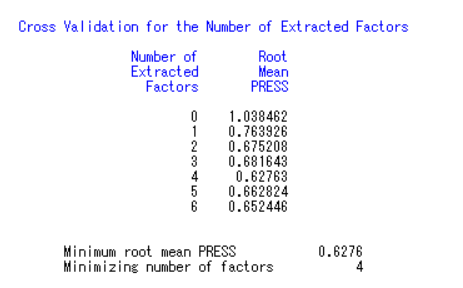提取4 个成分可使得PRESS 最小。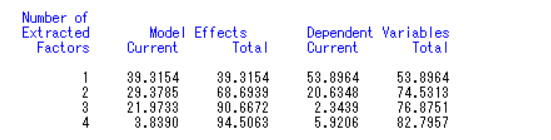提取的4 个成分解释自变量的比率为94.5063%，解释因变量的比率为82.7957%，说明建模效果较好。
原模型的参数估计：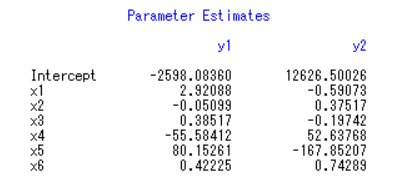根据分析结果，可得到原始变量的回归方程。
𝑦1 = −2598.08360 + 2.92088𝑥1 − 0.05099𝑥2 + 0.38517𝑥3 − 55.58412𝑥4 + 80.15261𝑥5 + 0.42225𝑥6
𝑦2 = 12626.50026 − 0.59073𝑥1 + 0.37517𝑥2 − 0.19742𝑥3 + 52.63768𝑥4 − 167.85207𝑥5 + 0.74289𝑥6
data ex;
input y1-y2 x1-x6@@;
y11=-2598.08360+2.92088*x1-0.05099*x2+0.38517*x3-55.58412*x4+80.15261*x5+0.42225*x6;
q1+(y1-y11)**2;
y21=12626.50026-0.59073*x1+0.37517*x2-0.19742*x3+52.63768*x4-167.85207*x5+0.74289*x6;
q2+(y2-y21)**2;
cards;
数据区
. . 114.81	5493	507.0	119.8	116.5	468.79
. . 47.76	5753	61.6	118.0	116.3	105.80
. . 61.98	5079	121.8	117.1	115.3	114.40
. . 376.95	5348	339.0	119.7	116.7	428.76
;
proc print;
var q1 q2 y11 y21;
run;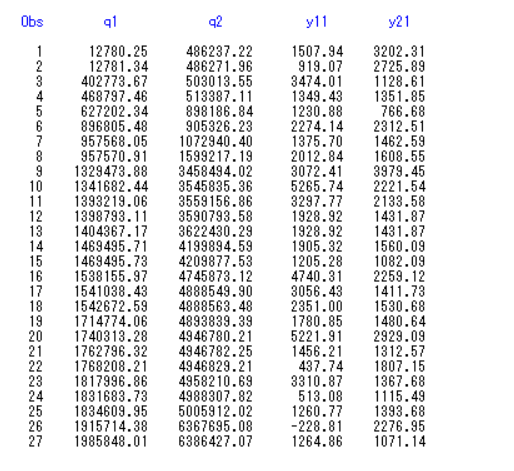对模型做残差检验，得到y1 和y2 的总残差分别为1985848.01、6386427.07，故此模型拟合不佳。
预测结果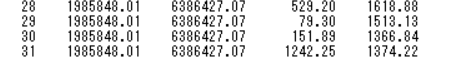结语
今晚IG打RNG，芜湖春晚又到了。我已经开始唱了你们呢：难忘今宵难忘今宵~~


展开全文机器学习 深度学习 数据挖掘 数学建模
• 讲述偏最小二乘直接求解，以及偏最小二乘的分类及应用
• 先用动态聚类法对福州市居民历史用电负荷进行聚类分析以获得一个样本空间，在此基础上采用偏最小二乘回归方法进行建模和短期负荷预测分析
• 论文研究-排水型深V型船形因子的偏最小二乘回归分析与建模.pdf, 采用偏最小二乘法对排水型深V型船的船形因子进行了回归分析与建模, 给出了回归公式的适用范围, 并与模型...
• 小二乘回归提供一种多对多线性回归建模的方法，特别当两组变量的个数很多，且都存在多重相关性，而观测数据的数量（样本量）又较少时，用小二乘回归建立的模型具有传统的经典回归分析等方法所没有的优点。...
给大家安利一款朋友开发的自研国产数据分析基础工具，一键式自动分析，自动生成分析模板，5分钟掌握主流61个统计类数学模型（几乎涵盖SPSS绝大部分功能），以及23个有监督机器学习（包括随机森林，SVM，XGBoost等）

PS：巨方便简单上手，貌似现在是免费

官网：www.mpaidata.com   mpai数据科学平台

偏小二乘回归提供一种多对多线性回归建模的方法，特别当两组变量的个数很多，且都存在多重相关性，而观测数据的数量（样本量）又较少时，用偏小二乘回归建立的模型具有传统的经典回归分析等方法所没有的优点。

偏小二乘回归分析在建模过程中集中了主成分分析，典型相关分析和线性回归分析方法的特点，因此在分析结果中，除了可以提供一个更为合理的回归模型外，还可以同时完成一些类似于主成分分析和典型相关分析的研究内容，提供更丰富、深入的一些信息。

例 本节采用兰纳胡德（Linnerud）给出的关于体能训练的数据进行偏小二乘回归建模。在这个数据系统中被测的样本点，是某健身俱乐部的20位中年男子。被测变量分为两组。第一组是身体特征指标X，包括：体重、腰围、脉搏。第二组变量是训练结果指标Y，包括：单杠、弯曲、跳高。原始数据见表1。

表2给出了这6个变量的简单相关系数矩阵。从相关系数矩阵可以看出，体重与腰围是正相关的；体重、腰围与脉搏负相关；而在单杠、弯曲与跳高之间是正相关的。从两组变量间的关系看，单杠、弯曲和跳高的训练成绩与体重、腰围负相关，与脉搏正相关。

表 1  体能训练数据

No

体重( )

腰围( )

脉搏( )

单杠( )

弯曲( )

跳高( )

1

191

36

50

5

162

60

2

189

37

52

2

110

60

3

193

38

58

12

101

101

4

162

35

62

12

105

37

5

189

35

46

13

155

58

6

182

36

56

4

101

42

7

211

38

56

8

101

38

8

167

34

60

6

125

40

9

176

31

74

15

200

40

10

154

33

56

17

251

250

11

169

34

50

17

120

38

12

166

33

52

13

210

115

13

154

34

64

14

215

105

14

247

46

50

1

50

50

15

193

36

46

6

70

31

16

202

37

62

12

210

120

17

176

37

54

4

60

25

18

157

32

52

11

230

80

19

156

33

54

15

225

73

20

138

33

68

2

110

43

均值

标准差

178.6

24.6905

35.4

3.202

56.1

7.2104

9.45

5.2863

145.55

62.5666

70.3

51.2775

表 2 相关系数矩阵

1

0.8702

-0.3658

-0.3897

-0.4931

-0.2263

0.8702

1

-0.3529

-0.5522

-0.6456

-0.1915

-0.3658

-0.3529

1

0.1506

0.225

0.0349

-0.3897

-0.5522

0.1506

1

0.6957

0.4958

-0.4931

-0.6456

0.225

0.6957

1

0.6692

-0.2263

-0.1915

0.0349

0.4958

0.6692

1

MATLAB源代码：

clc,clear
ab0=load('pz.txt');   %原始数据存放在纯文本文件pz.txt中
mu=mean(ab0);sig=std(ab0); %求均值和标准差
rr=corrcoef(ab0);   %求相关系数矩阵
ab=zscore(ab0); %数据标准化
a=ab(:,[1:3]);b=ab(:,[4:end]);  %提出标准化后的自变量和因变量数据
[XL,YL,XS,YS,BETA,PCTVAR,MSE,stats] =plsregress(a,b);
xw=a\XS;  %求自变量提出成分系数，每列对应一个成分，这里xw等于stats.W
yw=b\YS;  %求因变量提出成分的系数
a_0=PCTVAR(1,:);b_0=PCTVAR(2,:);
a_1=cumsum(a_0);b_1=cumsum(b_0);
i=1;%赋初始值
%判断提出成分对的个数
while ((a_1(i)<0.9)&(a_0(i)>0.05)&(b_1(i)<0.9)&(b_0(i)>0.05))
i=i+1;
end
ncomp=i;
fprintf('%d对成分分别为：\n',ncomp);
for i=1:ncomp
fprintf('第%d对成分：\n',i);
fprintf('u%d=',i);
for k=1:3%此处为变量x的个数
fprintf('+(%f*x_%d)',xw(k,i),k);
end
fprintf('\n');
fprintf('v%d=',i);
for k=1:3%此处为变量y的个数
fprintf('+(%f*y_%d)',yw(k,i),k);
end
fprintf('\n');
end
[XL2,YL2,XS2,YS2,BETA2,PCTVAR2,MSE2,stats2] =plsregress(a,b,ncomp);
n=size(a,2); m=size(b,2);%n是自变量的个数,m是因变量的个数
beta3(1,:)=mu(n+1:end)-mu(1:n)./sig(1:n)*BETA2([2:end],:).*sig(n+1:end); %原始数据回归方程的常数项
beta3([2:n+1],:)=(1./sig(1:n))'*sig(n+1:end).*BETA2([2:end],:); %计算原始变量x1,...,xn的系数，每一列是一个回归方程
fprintf('最后得出如下回归方程：\n')
for i=1:3%此处为变量y的个数
fprintf('y%d=%f',i,beta3(1,i));
for j=1:3%此处为变量x的个数
fprintf('+(%f*x%d)',beta3(j+1,i),j);
end
fprintf('\n');
end
bar(BETA2','k')   %画直方图
fprintf('因变量y的预测值：\n');
yhat=repmat(beta3(1,:),[size(a,1),1])+ab0(:,[1:n])*beta3([2:end],:);  %求y1,..,ym的预测值
disp(yhat);
ymax=max([yhat;ab0(:,[n+1:end])]); %求预测值和观测值的最大值
%下面画y1,y2,y3的预测图，并画直线y=x
figure, subplot(2,2,1)
plot(yhat(:,1),ab0(:,n+1),'*',[0:ymax(1)],[0:ymax(1)],'Color','k')
legend('单杠成绩预测图',2)
subplot(2,2,2)
plot(yhat(:,2),ab0(:,n+2),'O',[0:ymax(2)],[0:ymax(2)],'Color','k')
legend('弯曲成绩预测图',2)
subplot(2,2,3)
plot(yhat(:,3),ab0(:,end),'H',[0:ymax(3)],[0:ymax(3)],'Color','k')
legend('跳高成绩预测图',2)


该MATLAB源代码结果分析：根据PCTVAR可知，前两个成分解释自变量的比率为92.13%，只需要取两对成分即可。故ncomp的值应为2，beta3为最后求解出的回归方程。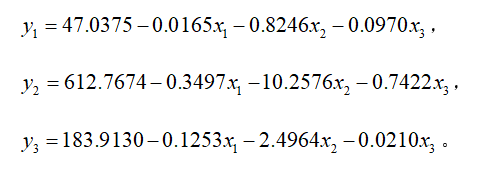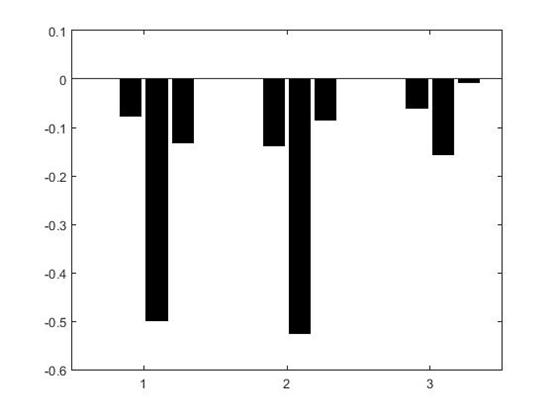图1 回归系数的直方图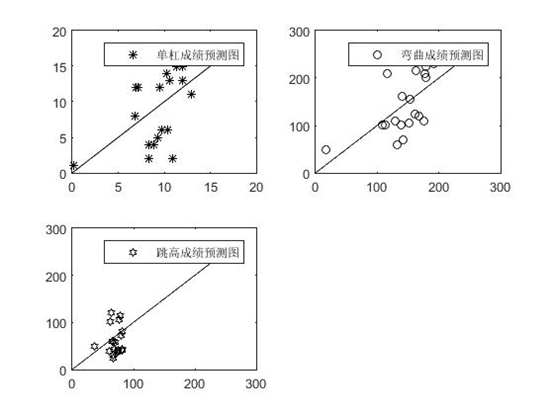展开全文• 在多种配置的计算机上对全球多地区多级别的三维地形进行漫游测试，得出实验数据，然后利用偏最小二乘回归方法，对三维可视化系统中影响系统性能的11个硬件因素进行了分析。实验结果表明，影响三维可视化系统的主要...
• 当两组变量的个数很多，且都存在多重相关性，而观测数据的数量（样本量）又较少时，用偏最小二乘回归建立模型 11.1 分析概述 在自变量集中提出第一成分u（尽可能多的提取原自变量中的变异信息），同时在因变量集中...数学建模
• 0.偏最小二乘回归集成了多元线性回归、主成分分析和典型相关分析的优点，在建模中是一个更好的选择，并且MATLAB提供了完整的实现，应用时主要的问题是： 注意检验，各种检验参数：有关回归的检验以及有关多元分析...
• PLS是一种可以处理多个因变量对多个自变量的回归建模方法。特别当各变量集合内部存在较高程度的相关性时,用PLS进行回归建模分析,比对逐个因变量做多元回归更加有效,其结论更加可靠,整体性更强
• 基础部数学教研室 基础部数学教研室 */49 数学建模 数学建模算法与应用 基础部数学教研室 基础部数学教研室 */49 数学建模
• 本文分析了目前应用一般的最小二乘法建立均匀试验数据的二次多项式回归模型时存在的局限性，提出了应用偏最小二乘法(Partial least-square，PLS)建立二次多项式回归模型的技术，并已在作者开发的统计分析软件(DPS...
• 1. 概述 2. matlab偏最小二乘回归命令 3. 案例分析 （略） 原课本习题及代码 点击下载 【课本 + 源码 】 提取码：92h8数学建模 算法
• 偏最小二乘回归系列博文： ...偏最小二乘回归分析建模的具体步骤 模型效应负荷量 交叉有效性检验 在实际问题中，经常遇到需要研究两组多重相关变量间的相互依赖关系，并研究用 一组变量（常称为自变量或预...
• lm(x0~t1) #验证α1即为x0做应变量，t1做自变量的最小回归的t1的回归系数(分别为weight、waist和pulse的回归系数，共3个) lm(y0~t1) #验证β1即为y0做应变量，t1做自变量的最小回归的t1的回归系数(分别为chins、...
• 偏最小二乘回归分析主要适用于多因变量对多自变量的回归建模，并可以有效地解决许多用普通多元回归无法解决的问题，诸如克服变量多重相关性在系统建模中的不良作用以及在样本容量小于变量个数的情况下进行回归建模等......